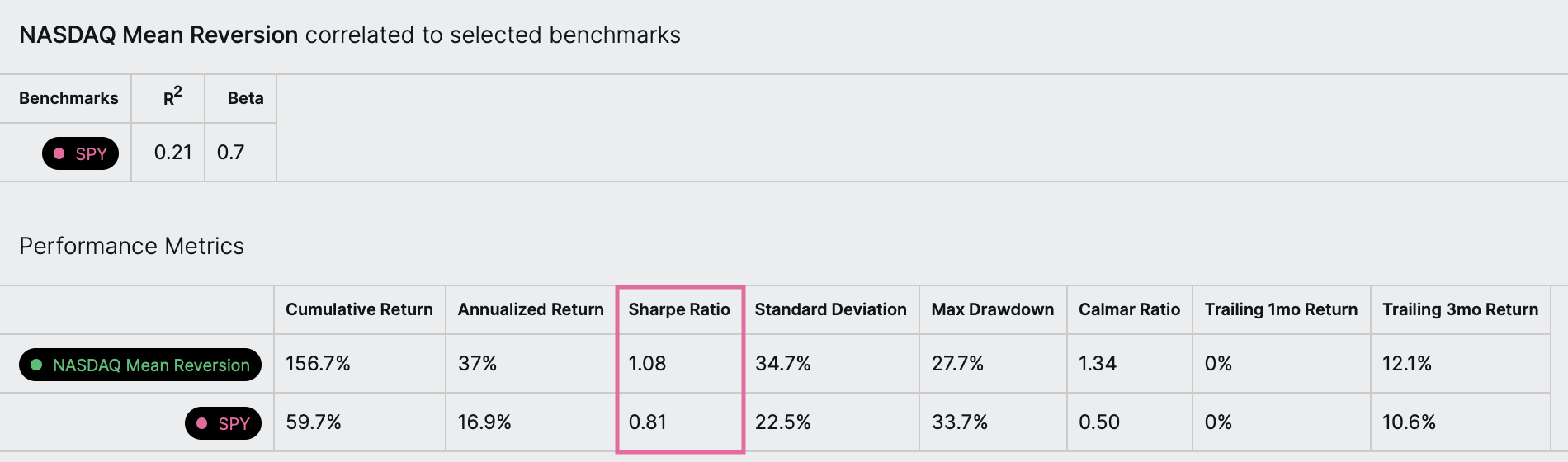# Sharpe ratio

What is it?

The Sharpe ratio is a measure of an investment's return adjusted for its risk (another measure of risk-adjusted return is the Calmar ratio). The higher the Sharpe ratio, the better a return an investment gets per unit of risk taken.

What is a “good” Sharpe ratio?

A Sharpe ratio over 1 is considered good, over 2, very good, and over 3, excellent.

An example

Let’s take a look at an example. In this backtest, we see the symphony’s Sharpe ratio is 1.08 while the benchmark’s (\$SPY) is 0.81. This means that the symphony had a better risk-adjusted return than the benchmark over the backtest time period.Traditional formula for the Sharpe ratio

The Sharpe ratio, developed by Nobel laureate William Sharpe, is traditionally calculated as follows: (Return of investment - Risk-free rate of return) / Standard deviation of the investment’s return in excess of the risk-free rate

The risk-free rate of return is the return of an investment with (hypothetically) zero risk, like the interest rate on three-month U.S. Treasury bills. The risk-free rate depends on what instrument is chosen (e.g., three-month or 10-year Treasury bills) and the time the investment is made, as the same instrument may perform differently at different times. In addition, using U.S. instruments to calculate the risk-free rate may not be appropriate for investors living in or investing in other countries.

For these reasons, Composer does not include the risk-free rate in the calculation of the Sharpe ratio. In other words, the risk-free rate is assumed to be zero.

How is the Sharpe ratio calculated in Composer?

The Sharpe ratio you see in Composer is calculated by dividing the annualized arithmetic mean of the daily returns by the annualized standard deviation of the daily returns. Both the return of the investment and its standard deviation are annualized -- they are standardized to a period of a year.

Why is the arithmetic mean of returns used in the calculation? The geometric mean is often used when calculating financial performance. The difference between the arithmetic mean and the geometric mean of returns is known as the volatility drag, because returns that vary over time have a lower geometric than arithmetic mean. The Sharpe ratio calculation corrects for this volatility drag by dividing the mean of the returns by the standard deviation of the returns (standard deviation is a measure of volatility). If the geometric mean were used in the numerator of the formula, the volatility drag would be corrected twice. While best practices are still up for debate, this practice could lead to overly-conservative estimates.

Step-by-step calculation of the Sharpe ratio

Let's go through the calculation of the Sharpe ratio step-by-step for a symphony backtest. Here's how we do it:

For the numerator (Annualized arithmetic mean of the daily returns):

• Compute each day’s percent return over the backtesting period
• Compute the arithmetic mean of those daily returns (i.e., sum them and divide by the number of values, which is the number of trading days in the backtest)
• Annualize that value, which in this case means multiply by 252, the typical number of trading days in a year.

For the denominator (Annualized standard deviation of the daily returns):

• Compute each day’s percent return over the backtesting period
• Compute the standard deviation of those daily returns
• Annualize that value, which in this case means multiplying by the square root of 252, the typical number of trading days in a year.

Divide the annualized arithmetic mean of the daily returns by the annualized standard deviation of the daily returns.